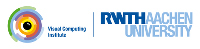OpenMesh
Filtering ranges with predicates

This examples shows:

• How to use predicates to filter which elements of a mesh you want iterate over

In the previous tutorial we discussed already that the ranges returned by functions like all_vertices(), voh_range() or outgoing_halfedges() provide a few helpful methods such as avg() or to_vector(). Another interesting method is filtered() which requires as argument something that can be called for an element of the range and returns a bool. The resulting range will then only iterate over elements for which the filter returs true. The filter can be a lambda, a function pointer, a property manager holding a bool property, or a functor object such as the predicates defined in <OpenMesh/Core/Utils/Predicates.hh>. The predefined predicates can check the status of a mesh element and test if they are boundary. With their help you can for example count all boundary vertices:

// Count boundary vertices
std::cout << "Mesh contains " << mesh.vertices().count_if(Boundary()) << " boundary vertices";

Predicates can be composed using the operators ||, &&, and !. This enables you to specify precisely which elements you want process in your loop, e.g. inner vertices that are selected:

// Selected inner vertices
std::cout << "These are the selected inner vertices: " << std::endl;
for (auto vh : mesh.vertices().filtered(!Boundary() && Selected()))
std::cout << vh.idx() << ", ";
std::cout << std::endl;

As mentioned above, the filter argument can be anything returning a bool for a given input, e.g. a function pointer:

// Faces whose id is divisible by 3
auto vec = mesh.faces().filtered(is_divisible_by_3).to_vector();
std::cout << "There are " << vec.size() << " faces whose id is divisible by 3" << std::endl;

However, function pointers, lambdas etc are not composable. Fortunately, you can use make_predicate to turn them into composable predicates:

// Faces which are tagged or whose id is not divisible by 3
auto vec2 = mesh.faces().filtered(Tagged() || !make_predicate(is_divisible_by_3)).to_vector();
std::cout << "There are " << vec2.size() << " faces which are tagged or whose id is not divisible by 3" << std::endl;

Below is the complete source code:

#include <OpenMesh/Core/IO/MeshIO.hh>
#include <OpenMesh/Core/Mesh/DefaultTriMesh.hh>
#include <OpenMesh/Core/Utils/PropertyManager.hh>
#include <OpenMesh/Core/Utils/Predicates.hh>
#include <iostream>
#include <vector>
bool is_divisible_by_3(OpenMesh::FaceHandle vh) { return vh.idx() % 3 == 0; }
int main(int argc, char** argv)
{
MyMesh mesh;
if (argc != 4) {
std::cerr << "Usage: " << argv << " infile" << std::endl;
return 1;
}
const std::string infile = argv;
std::cerr << "Error: Cannot read mesh from " << infile << std::endl;
return 1;
}
// Count boundary vertices
std::cout << "Mesh contains " << mesh.vertices().count_if(Boundary()) << " boundary vertices";
// Selected inner vertices
std::cout << "These are the selected inner vertices: " << std::endl;
for (auto vh : mesh.vertices().filtered(!Boundary() && Selected()))
std::cout << vh.idx() << ", ";
std::cout << std::endl;
// Faces whose id is divisible by 3
auto vec = mesh.faces().filtered(is_divisible_by_3).to_vector();
std::cout << "There are " << vec.size() << " faces whose id is divisible by 3" << std::endl;
// Faces which are tagged or whose id is not divisible by 3
auto vec2 = mesh.faces().filtered(Tagged() || !make_predicate(is_divisible_by_3)).to_vector();
std::cout << "There are " << vec2.size() << " faces which are tagged or whose id is not divisible by 3" << std::endl;
// Edges that are longer than 10 or shorter than 2
OpenMesh::EProp<bool> longer_than_10(mesh);
for (auto eh : mesh.edges())
longer_than_10[eh] = mesh.calc_edge_length(eh) > 10;
std::cout << "There are " <<
mesh.edges().count_if(make_predicate(longer_than_10) || make_predicate([&](OpenMesh::EdgeHandle eh) { return mesh.calc_edge_length(eh) < 2; })) <<
" edges which are shorter than 2 or longer than 10" << std::endl;
}
bool read_mesh(Mesh &_mesh, const std::string &_filename)
Read a mesh from file _filename.
Definition: MeshIO.hh:95
int idx() const
Get the underlying index of this handle.
Definition: Handles.hh:69
Handle for a edge entity.
Definition: Handles.hh:135
Handle for a face entity.
Definition: Handles.hh:142
Triangle mesh based on the ArrayKernel.
Definition: TriMesh_ArrayKernelT.hh:96
Polygonal mesh based on the ArrayKernel.
Definition: PolyMesh_ArrayKernelT.hh:96
Scalar calc_edge_length(EdgeHandle _eh) const
Compute normals for all primitives.
Definition: PolyMeshT.hh:424
This class is intended to manage the lifecycle of properties.
Definition: PropertyManager.hh:76

Project OpenMesh, ©  Visual Computing Institute, RWTH Aachen. Documentation generated using doxygen .